## Example Questions

### Example Question #1 : Right Triangles

The measure of 3 angles in a triangle are in a 1:2:3 ratio. What is the measure of the middle angle?

45
90
30
60
Explanation:

The angles in a triangle sum to 180 degrees. This makes the middle angle 60 degrees.

### Example Question #1 : How To Find An Angle In A Right Triangle

A 17 ft ladder is propped against a 15 ft wall. What is the degree measurement between the ladder and the ground?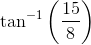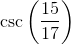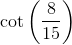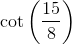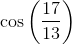Explanation:

Since all the answer choices are in trigonometric form, we know we must not necessarily solve for the exact value (although we can do that and calculate each choice to see if it matches). The first step is to determine the length of the ground between the bottom of the ladder and the wall via the Pythagorean Theorem: "x+ 152 = 172"; x = 8. Using trigonometric definitions, we know that "opposite/adjecent = tan(theta)"; since we have both values of the sides (opp = 15 and adj = 8), we can plug into the tangential form tan(theta) = 15/8. However, since we are solving for theta, we must take the inverse tangent of the left side, "tan-1". Thus, our final answer is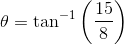### Example Question #51 : Plane Geometry

What is the sine of the angle between the base and the hypotenuse of a right triangle with a base of 4 and a height of 3?Explanation:

By rule, this is a 3-4-5 right triangle. Sine = (the opposite leg)/(the hypotenuse). This gives us 3/5.

### Example Question #3 : How To Find An Angle In A Right Triangle

In a right triangle ABC, the measure of angle C is greater than 60 degrees. Which of the following statements could describe the measures of angles A and B?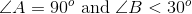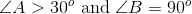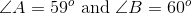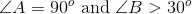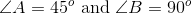Explanation:

Given that it is a right triangle, either angle A or B has to be 90 degrees. The other angle then must be less than 30 degrees, given that C is greater than 60 because there are 180 degrees in a triangle.

Example:

If angle C is 61 degrees and angle A is 90 degrees, then angle B must be 29 degrees in order for the angle measures to sum to 180 degrees.

### Example Question #4 : How To Find An Angle In A Right Triangle

Right triangle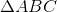has angles with a ratio of with a ratio of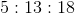. What is the smallest angle in the triangle?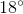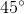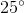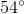Explanation:

Solving this problem quickly requires that we recognize how to break apart our ratio.

The Triangle Angle Sum Theorem states that the sum of all interior angles in a triangle is. Additionally, the Right Triangle Acute Angle Theorem states that the two non-right angles in a right triangle are acute; that is to say, the right angle is always the largest angle in a right triangle.

Since this is true, we can assume thatis represented by the largest number in the ratio of angles. Now consider that the other two angles must also sum to. We know therefore that the sum of their ratios must be divisible byas well.

Thus,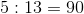.

To find the value of one angle of the ratio, simply assign fractional value to the sum of the ratios and multiply by.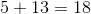, so: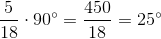Thus, the shortest angle (the one represented byin our ratio of angles) is.

### Example Question #5 : How To Find An Angle In A Right Triangle

Right trianglehas an acute angle measuring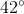. What is the measure of the other acute angle?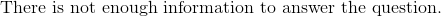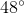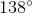Explanation:

The Triangle Angle Sum Theorem states that the sum of all interior angles in a triangle must be. We know that a right triangle has one angle equal to, and we are told one of the acute angles is.

The rest is simple subtraction: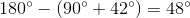Thus, our missing angle is.